Author: Oscar Cronquist Article last updated on October 15, 2019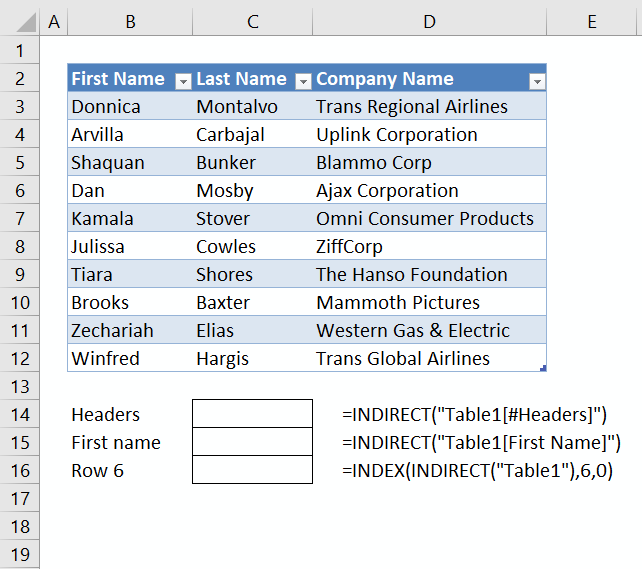This article demonstrates different ways to reference an Excel defined Table in a drop-down list and Conditional Formatting. There are two approaches, the INDIRECT function or a named range.

The INDIRECT function is a volatile function meaning it recalculates every time Excel recalculates which is not the case with most Excel functions. If used a lot the INDIRECT function may slow down your workbook calculations considerably, be careful.

It is enough to calculate a formula once, there is no need to calculate all cells every time Excel recalculates. This makes your worksheets faster and more efficient, however, this is not the case with a handful of functions and the INDIRECT function is one of them.

Another downside with the INDIRECT function apart from being volatile is that the Excel Table name is "hardcoded" into the formula. The formula will stop working if you change the Excel Table name.

#### How to populate a drop-down list with Excel Table headers?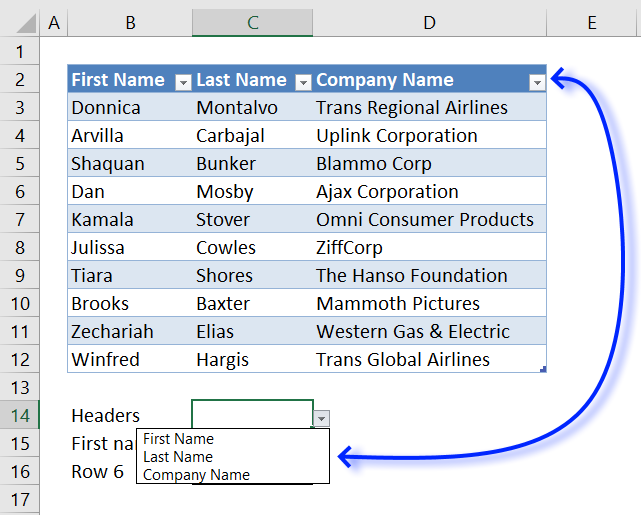The image above shows a drop-down list populated with Excel Table header values, this formula allows you to use Excel table headers as values in a drop-down list.

You can also create a named range and reference the headers there.

1. Go to tab "Formulas" on the ribbon.
2. Press with left mouse button on "Name Manager" button to open the "Name Manager" dialog box.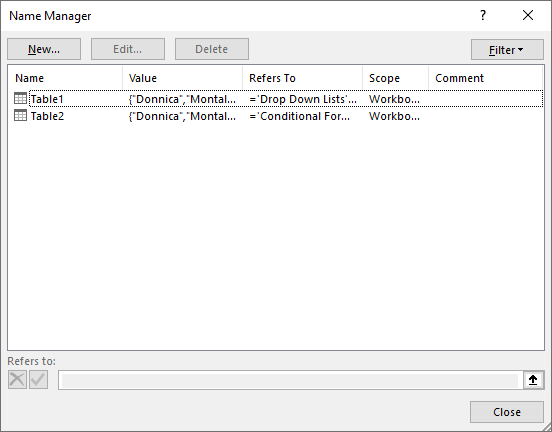3. Press with left mouse button on the "New" button.
4. Type the reference, in this case: =Table1[#Headers]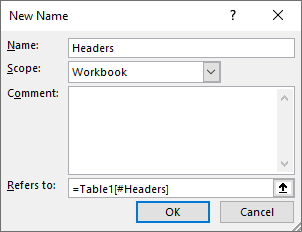5. Press with left mouse button on OK button.
6. Press with left mouse button on Close button.

Now use the named range name Headers in the Data Validation dialog box.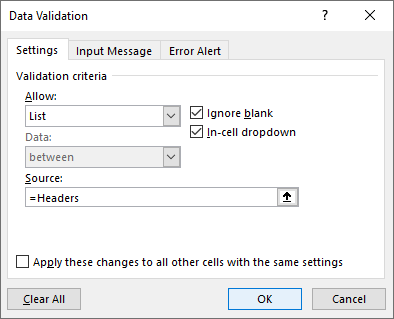#### How to populate a drop-down list with values from an Excel Table column?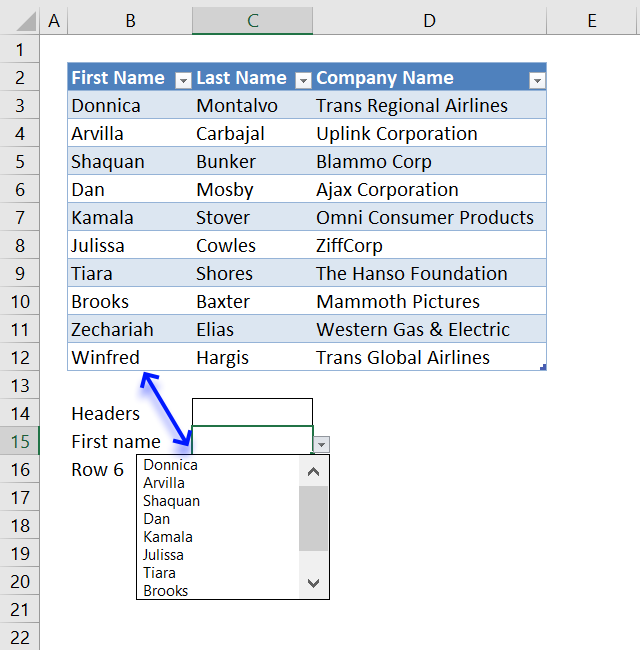The easiest way is to use the INDIRECT function to create a reference to an Excel Table column. References pointing to an Excel Table are called "structured references", they are different from regular cell references.

Cell B15 contains a drop-down list with this formula:

=INDIRECT("Table1[First Name]")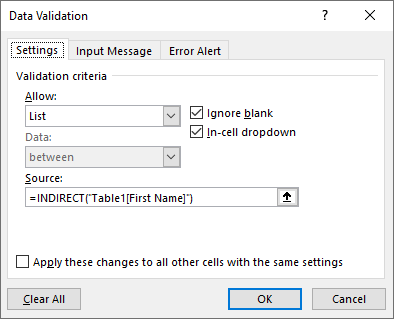#### How to populate a drop-down list with values from an Excel Table row?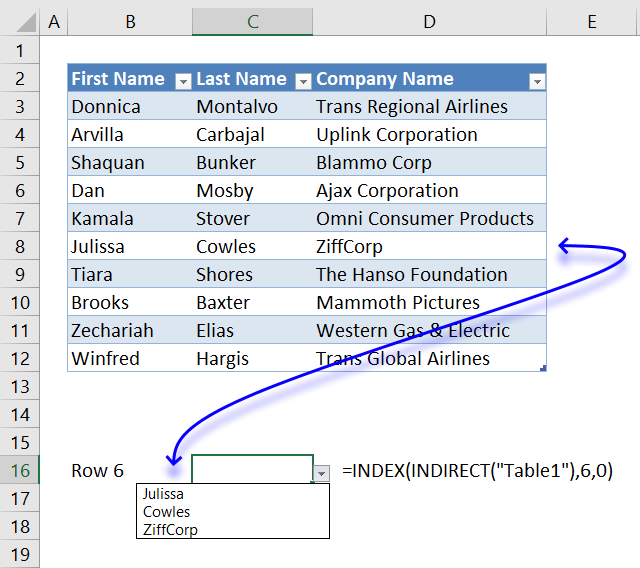The INDEX function is capable of extracting values in a single row from a cell range, combine it with the INDIRECT function and a reference to the Excel Table, please see formula below.

Reference a table row in a drop down list, cell B15:

=INDEX(INDIRECT("Table1"),6,0)

#### Animated image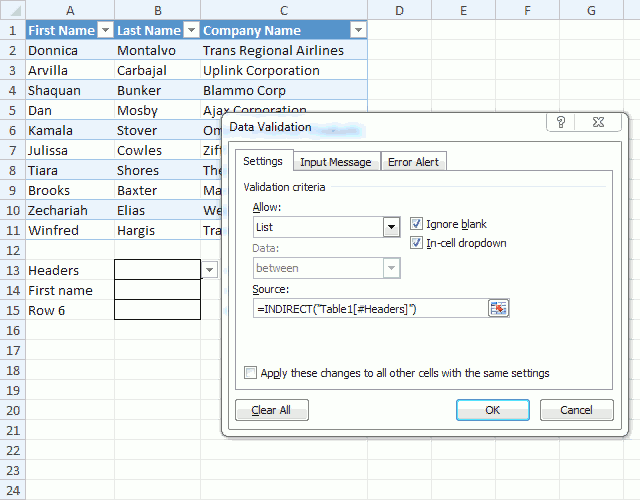The animated image above demonstrates the drop-down lists, how they work and what they link to.

#### How to reference an Excel Table in a Conditional Formatting formula?Simply add the INDIRECT function to each Excel Table reference in your formula, please see formula below.

Conditional formatting formula, cell range A13:C22:

=COUNTIFS(INDIRECT("Table2[First Name]"),\$A13,INDIRECT("Table2[Last Name]"),\$B13,INDIRECT("Table2[Company Name]"),\$C13)

The Conditional Formatting formula highlights a row if a record in cell range A13:C22 is equal to at least one record in the Excel defined Table (A1:C11)

### Get the Excel fileReference-a-table-in-a-data-validation-list-or-conditional-formatting-formula.xlsx